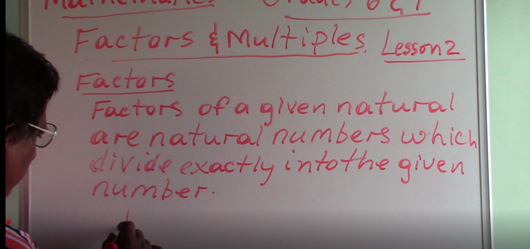# Grade 6 & 7 - Factors (Introduction)

Regular price \$2.00 Sale

A short five (5) minute Math Tutorial on Factors (Grade 6 and 7). Lecture done by M. Chan (Trained Certified Teacher).

This is our second lesson and it is based on Factors and Multiples. Factors of a given Natural Number are Natural Numbers which divide exactly into the given Number. Now lets say we have the Natural Number 12. Factors of 12, will be....you can write this as 12 subscript F. These will be 1, which is a factor of any Natural Number....